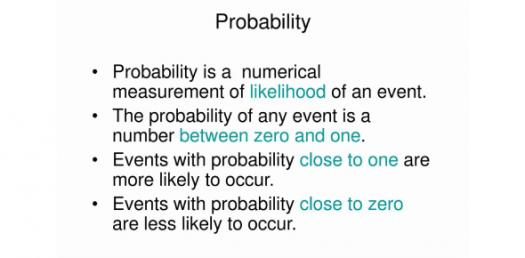Math: Test Your Probability Knowledge! Quiz

24 Questions | Total Attempts: 377SettingsProbability is significant for our daily lives. Statistical analysis is based on probability. You can utilize statistics to work out the likelihood that a specific event will occur. When you calculate the probability of an event, you look at the probability of the desired outcomes versus everything that can occur. Then, take this quiz to test what you know about probability.

• 1.
"Probability" is defined as:
• A.

Favorable outcomes divided by unfavorable outcomes

• B.

Favorable outcomes divided by total outcomes

• C.

Total outcomes divided by favorable outcomes

• D.

Unfavorable outcomes divided by favorable outcomes

• 2.
"Odds" are defined as:
• A.

Favorable outcomes divided by unfavorable outcomes

• B.

Favorable outcomes divided by total outcomes

• C.

Total outcomes divided by favorable outcomes

• D.

Unfavorable outcomes divided by favorable outcomes

• 3.
Probabilities can be written using:
• A.

Fractions

• B.

Decimals

• C.

Percents

• D.

Values greater than 1

• E.

Values between 0 and 1

• F.

Values less than 0

• 4.
Theoretical probability:
• A.

Is based on mathematical rules.

• B.

Is the result of an experiment.

• C.

Is less accurate than than "odds"

• 5.
Experimental probability:
• A.

Compares favorable outcomes to unfavorable outcomes.

• B.

Demonstrates the number of times that an event actually occurred.

• C.

Becomes less accurate the more that the experiment is repeated

• 6.
Suppose that you have a bag containing 3 red jelly beans, 4 green jelly beans, 5 yellow jelly beans, and 1 black jelly bean.  If you pick one jelly bean from the bag at random, what is the probability that the bean will be yellow?
• 7.
Suppose that you have a bag containing 3 red jelly beans, 4 green jelly beans, 5 yellow jelly beans, and 1 black jelly bean.What are the odds against drawing a green jelly bean?
• 8.
Suppose that you have a bag containing 3 red jelly beans, 4 green jelly beans, 5 yellow jelly beans, and 1 black jelly bean. If you pick one jelly bean, what is the probability that it will be either red or green?
• 9.
Suppose that you have a bag containing 3 red jelly beans, 4 green jelly beans, 5 yellow jelly beans, and 1 black jelly bean.If you pick a jelly bean, observe its color and return it to the bag, what is the chance that you will pick 3 red jelly beans in a row?
• 10.
Suppose that you have a bag containing 3 red jelly beans, 4 green jelly beans, 5 yellow jelly beans, and 1 black jelly bean.What is the probability that you will draw a purple jelly bean?
• 11.
What is probability?
• A.

A way of counting objects in a space

• B.

A way of expressing knowledge or belief that an event will occur or has occurred

• C.

A way for people to predict if something will happen

• 12.
The menu at a restaurant has 3 drinks, 4 appetizers, and 5 main courses.  How many different meals are possible?
• A.

12

• B.

15

• C.

60

• 13.
On a game show, the game that a contestant plays is chosen by spinning a spinner.  The spinner has 5 colors on it:  red, green, blue, purple, and orange.   What is the complement of spinning a red or orange on this spinner? (Remember: complement = NOT)
• A.

Purple

• B.

Red, green, purple, orange, blue

• C.

Green, purple, blue

• 14.
John has a bag of marbles.  In the bag, there are 4 yellow marbles, 7 red marbles, and 3 green marbles.  What is the probability that John will pull out a yellow marble?
• 15.
When a die is rolled, what is the complement of getting an odd number?
• A.

Getting an even number

• B.

Getting an odd number

• C.

Getting a 4 or 5

• 16.
There are 20 girls and 35 boys in the lunch room.  What is the probability of choosing a boy to sit with?
• 17.
There are 52 cards in a deck.  What is the probability of choosing a jack?
• 18.
There are 10 pencils, 3 pens, and 14 markers in a bin.  What is the probability of choosing a pen and a pencil?
• A.

3/729

• B.

10/27

• C.

30/729

• D.

13/27

• 19.
There are 52 cards in a deck.  What is the probability of choosing a jack and a king?
• A.

16/2704

• B.

52/2704

• C.

4/52

• D.

8/52

• 20.
There are 34 kittens, 12 dogs, and 15 birds at a pet store.  What is the probability that a customer will purchase a dog?
• 21.
A die is rolled. What is the complement of rolling a 5?
• A.

Rolling a 1, 2, 3, 4, or 5

• B.

Rolling a 1, 2, 3, or 4

• C.

Rolling a 5

• 22.
There are 6 pink, 2 blue, and 8 green socks in a drawer.  What is the probability of the complement of picking a pink sock? (Remember: complement = NOT)
• A.

6/16

• B.

2/16

• C.

10/16

• 23.
Jason has a bag full of coins.  There are 12 dimes, 21 pennies, 13 nickels, and 7 quarters in his bag.  If he chooses a coin at random, what is the probability that he chooses either a quarter and a dime?
• A.

84/2809

• B.

12/53

• C.

19/2809

• D.

19/53

• 24.
Sarah has 4 tomatoes, 3 potatoes, and 5 apples in her bag.  What is the probability of the complement of her eating a tomato? (Remember: complement = NOT)
• A.

8/12

• B.

4/12

• C.

5/12

Related TopicsBack to top